# Optoelectronics

LEDs, Lasers, Detectors - Zinc blende and wurtzite materials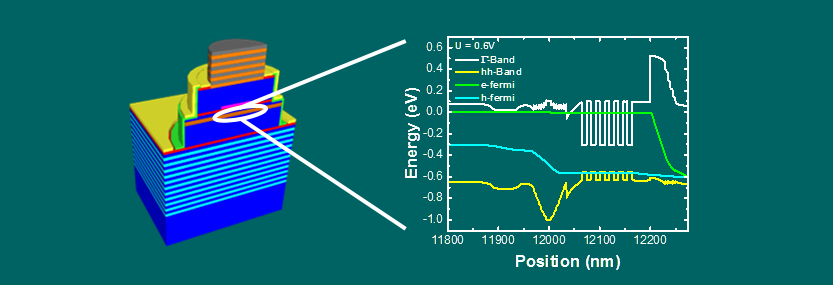## Tutorials## Quantum Dot Tutorials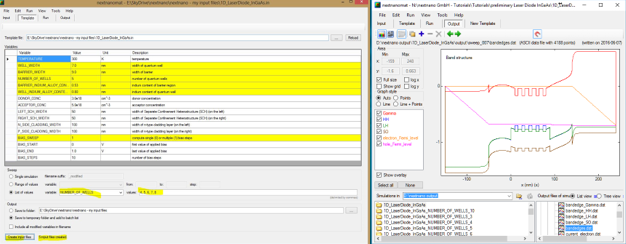## Laser Diode (InGaAs)

What you can learn:
• drift-diffusion current based on quantum density, pn junction, quaternaries, emission spectrum
• self-consistent Schrödinger-Poisson-Current equation in 1D

## AlGaInP on GaAsWhat you can learn:
• alloys, linearly graded alloys, bowing, strain, band gaps
• strain equation

## Wurtzite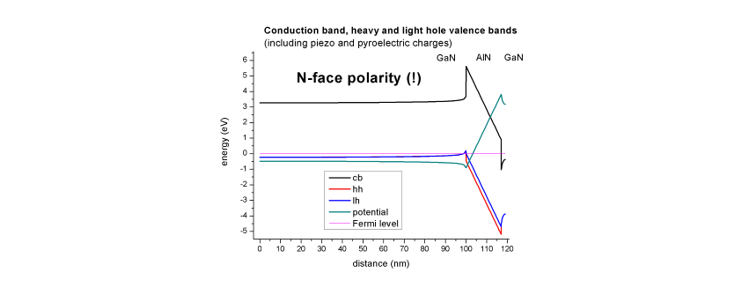What you can learn:
• wurtzite, strain, piezoelectricity, pyroelectricity
• strain equation, Poisson equation## Core-shell nanowires

What you can learn:
• 2D simulations, circular and hexagonal quantum confinement, core-shell nanowires, sweep over alloy concentrations and core radius, interband transitions (spatial overlap matrix elements)
• Schrödinger equation in 2D, self-consistent Schrödinger-Poisson equation in 2D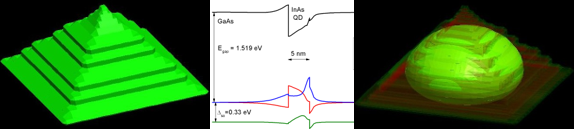## Quantum dot (pyramid)

What you can learn:
• 3D simulation, quantum dot, strain, piezoelectric fields
• Strain equation, Poisson equation, Schrödinger equation in 3D

## Strained substrate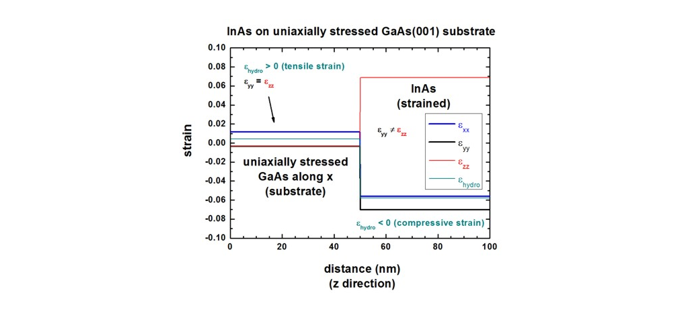What you can learn:
• uniaxial and biaxial strain, stress
• strain equation

## Broken gap quantum well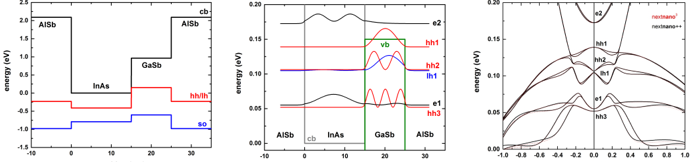InAs / GaSb broken gap quantum well (BGQW) (type-II band alignment)

What you can learn:
• 1D simulation, 8-band k·p, broken gap, type-II band alignment
• 8-band k·p equation

## Optical absorption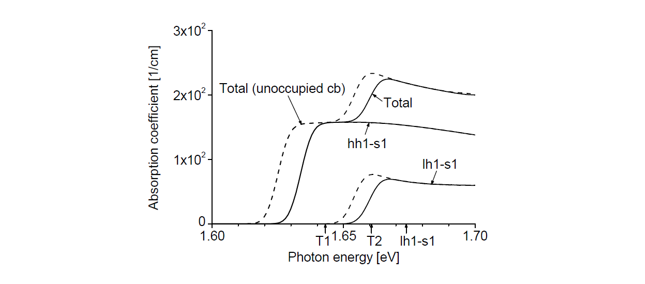Optical absorption of a quantum well - Diploma thesis documentation available

What you can learn:
• 8-band k·p, optical absorption
• 8-band k·p equation